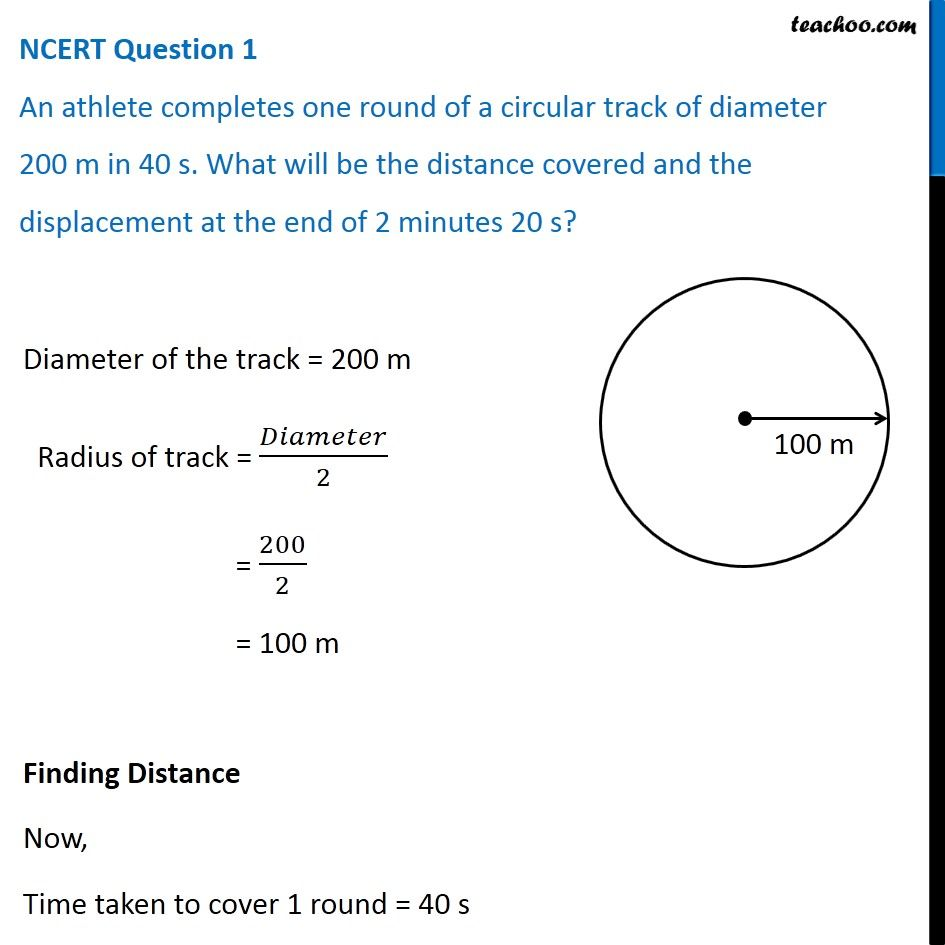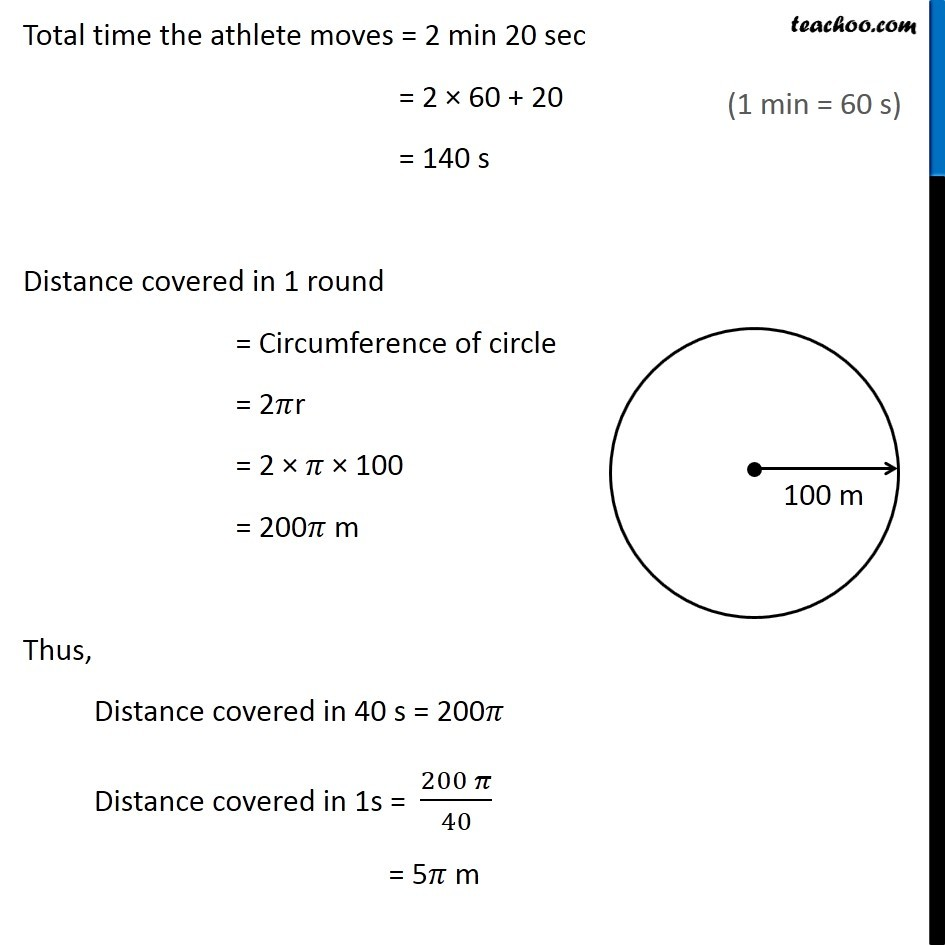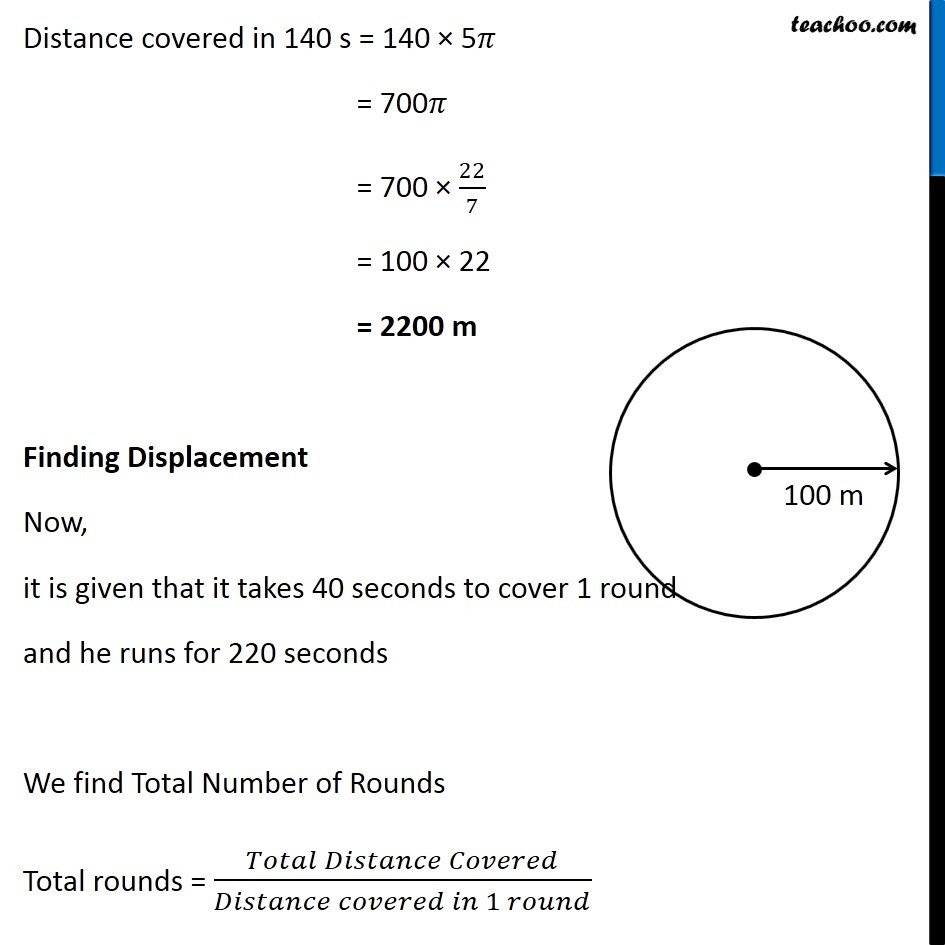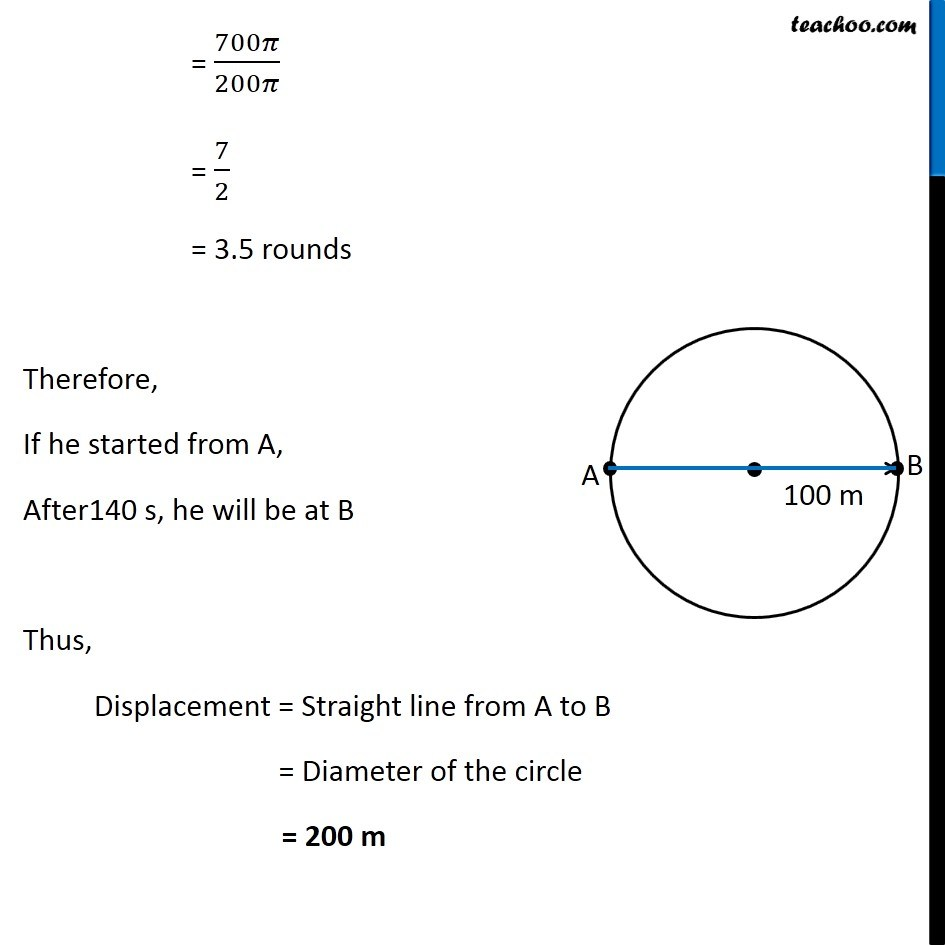NCERT Questions

Class 9
Chapter 8 Class 9 - MotionLearn in your speed, with individual attention - Teachoo Maths 1-on-1 Class

### Transcript

NCERT Question 1 An athlete completes one round of a circular track of diameter 200 m in 40 s. What will be the distance covered and the displacement at the end of 2 minutes 20 s? Diameter of the track = 200 m Radius of track = 𝐷𝑖𝑎𝑚𝑒𝑡𝑒𝑟/2 = 200/2 = 100 m Finding Distance Now, Time taken to cover 1 round = 40 s Total time the athlete moves = 2 min 20 sec = 2 × 60 + 20 = 140 s Distance covered in 1 round = Circumference of circle = 2𝜋r = 2 × 𝜋 × 100 = 200𝜋 m Thus, Distance covered in 40 s = 200𝜋 Distance covered in 1s = (200 𝜋)/40 = 5𝜋 m Distance covered in 140 s = 140 × 5𝜋 = 700𝜋 = 700 × 22/7 = 100 × 22 = 2200 m Finding Displacement Now, it is given that it takes 40 seconds to cover 1 round and he runs for 220 seconds We find Total Number of Rounds Total rounds = (𝑇𝑜𝑡𝑎𝑙 𝐷𝑖𝑠𝑡𝑎𝑛𝑐𝑒 𝐶𝑜𝑣𝑒𝑟𝑒𝑑)/(𝐷𝑖𝑠𝑡𝑎𝑛𝑐𝑒 𝑐𝑜𝑣𝑒𝑟𝑒𝑑 𝑖𝑛 1 𝑟𝑜𝑢𝑛𝑑) = 700𝜋/200𝜋 = 7/2 = 3.5 rounds Therefore, If he started from A, After140 s, he will be at B Thus, Displacement = Straight line from A to B = Diameter of the circle = 200 m